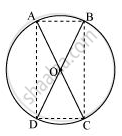# AC and BD are chords of a circle which bisect each other. Prove that (i) AC and BD are diameters; (ii) ABCD is a rectangle. - Mathematics

AC and BD are chords of a circle which bisect each other. Prove that (i) AC and BD are diameters; (ii) ABCD is a rectangle.

#### SolutionLet two chords AB and CD are intersecting each other at point O.

In ΔAOB and ΔCOD,

OA = OC (Given)

OB = OD (Given)

∠AOB = ∠COD (Vertically opposite angles)

ΔAOB ≅ ΔCOD (SAS congruence rule)

AB = CD (By CPCT)

Similarly, it can be proved that ΔAOD ≅ ΔCOB

∴ AD = CB (By CPCT)

Since in quadrilateral ACBD, opposite sides are equal in length, ACBD is a parallelogram.

We know that opposite angles of a parallelogram are equal.

∴ ∠A = ∠C

However, ∠A + ∠C = 180° (ABCD is a cyclic quadrilateral)

⇒ ∠A + ∠A = 180°

⇒ 2 ∠A = 180°

⇒ ∠A = 90°

As ACBD is a parallelogram and one of its interior angles is 90°, therefore, it is a rectangle.

∠A is the angle subtended by chord BD. And as ∠A = 90°, therefore, BD should be the diameter of the circle. Similarly, AC is the diameter of the circle.

Is there an error in this question or solution?
Chapter 10: Circles - Exercise 10.6 [Page 186]

#### APPEARS IN

NCERT Class 9 Maths
Chapter 10 Circles
Exercise 10.6 | Q 7 | Page 186

Share